International
Tables for
Crystallography
Volume B
Reciprocal space
Edited by U. Shmueli

International Tables for Crystallography (2006). Vol. B, ch. 3.4, p. 390   | 1 | 2 |

## Section 3.4.9. Evaluation of the incomplete gamma function

D. E. Williamsa

aDepartment of Chemistry, University of Louisville, Louisville, Kentucky 40292, USA

### 3.4.9. Evaluation of the incomplete gamma function

| top | pdf |

The incomplete gamma function may be expressed in terms of commonly available functions such as the exponential integral and the complement of the error function. The definition of the exponential integral is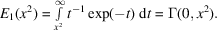The definition of the complement of the error function is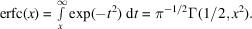Numerical approximations to these functions are given, for example, by Hastings (1955). The recursion formula for the incomplete gamma function (Davis, 1972)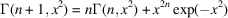may be used to obtain working formulae starting from the special values of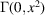and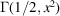which are defined above. Also we note that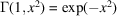.

### References

Davis, P. J. (1972). Gamma function and related functions. Handbook of mathematical functions with formulas, graphs, and mathematical tables, edited by M. Abramowitz & I. A. Stegun, pp. 260–262. London, New York: John Wiley. [Reprint, with corrections of 1964 Natl Bur. Stand. publication.]
Hastings, C. Jr (1955). Approximations for digital computers. New Jersey: Princeton University Press.